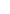## Microprocessors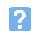1. In the microprocessor system shown below which feature represents the central processing unit (CPU)?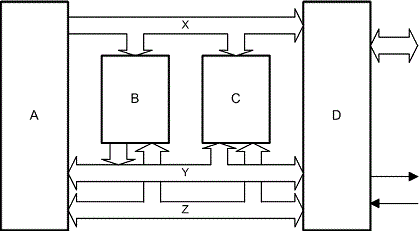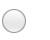a) Ab) Bc) Cd) D.2. In the microproceossor system shown, the feature marked X is the:a) address busb) control busc) data busd) I/O bus.3. Data that is retained when the power is removed from a semiconductor memory device is referred to as:a) program datab) transient datac) working datad) non-volatile data.4. What is the function of the circuit shown below?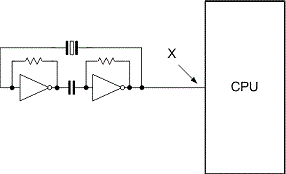a) Clockb) I/Oc) Memoryd) Reset.5. In the circuit shown, the signal appearing at point X will be:a) a triangle wave at a frequency of 50Hz or 100Hzb) a series of irregular pulses representing a stream of datac) a sine wave at a frequency of typically between 1kHz and 1MHzd) a square wave at a frequency of typically between 4MHz and 20 MHz.6. Which one of the following gives the function of the circuit shown below?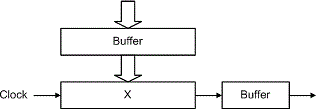a) Providing an accurate clock signalb) Converting parallel data into serial datac) Converting serial data into parallel datad) Generating regular interrupt signals for timing purposes.7. In the circuit shown, the feature marked X is:a) a clock circuitb) a memory devicec) a binary counterd) a shift register.8. The largest data value that can appear on an 8-bit data bus at any instant of time is equivalent to the decimal number:a) 7b) 8c) 15d) 255.9. The binary data 10100111 is equivalent to the hexadecimal value:a) 7Ab) A7c) 107d) 227.10. The outcome of an ALU operation, such as a zero or overflow condition, is held in the:a) accumulatorb) stack pointerc) instruction registerd) flag or status register.
This is more feedback!
This is the feedback!